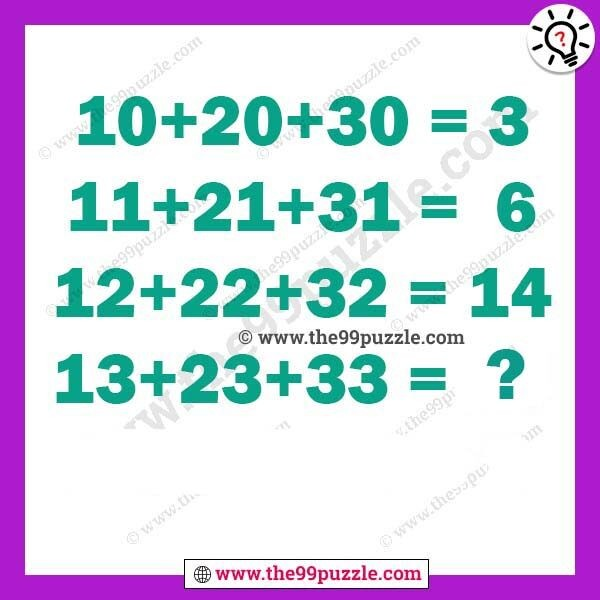# Tricky math puzzles with answer – Puzz189

Tricky math puzzles with answer. Simple math logic equation can solve both kids and adults all grade students. 99% of people fail to answer this simple math logic equation. If you are intelligent people you can easily solve these puzzles?

10+20+30=3

11+21+31=6

12+22+32=14

13+23+33=?###### Explanation:

10+20+30 = 1° + 2° + 3° = 1+1+1 = 3

11+21+31= 1¹ + 2¹ + 3¹ = 1+2+3 = 6

12+22+32= 1² + 2² + 3² = 1+4+9 = 14

13+23+33= 1³+2³+3³ = 1+8+27 = 36